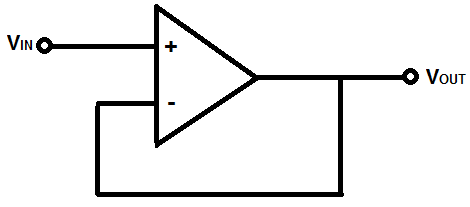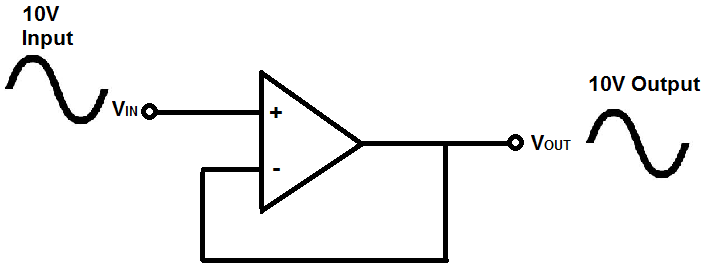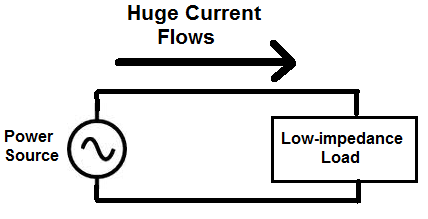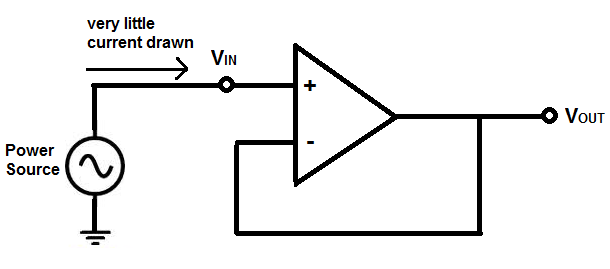﻿ What is a Unity Gain Buffer?# What is a Unity Gain Buffer?A unity gain buffer (also called a unity-gain amplifier) is a op-amp circuit which has a voltage gain of 1.

This means that the op amp does not provide any amplification to the signal. The reason it is called a unity gain buffer (or amplifier) is because it provides a gain of 1, meaning there is no gain; the output voltage signal is the same as the input voltage. Thus, for example, if 10V goes into the op amp as input, 10V comes out as output. A unity gain buffer acts as a true buffer, providing no amplification or attenuation to the signal.### What is the Purpose of a Unity Gain Buffer?

One may ask then, what is the purpose of a unity gain buffer? Since it outputs the same signal it inputs, what is its purpose in a circuit? This will now be explained.

An op amp circuit is a circuit with a very high input impedance. This high input impedance is the reason unity gain buffers are used. This will now be explained.

When a circuit has a very high input impedance, very little current is drawn from the circuit. If you know ohm's law, you know that current, I=V/R. Thus, the greater the resistance, the less current is drawn from a power source. Thus, the power of the circuit isn't affected when current is feeding a high impedance load.

Let's look at both illustrations below:

The below circuit is a circuit in which a power source feeds a low-impedance load.In this circuit above, the load demands and draws a huge amount of current, because the load is low impedance. According to ohm's law, again, current, I=V/R. If a load has very low resistance, it draws huge amounts of current. This causes huge amounts of power to be drawn by the power source and, because of this, causes high disturbances and use of the power source powering the load.

Now let's look at the circuit below, connected to a unity-gain bufffer:This circuit above now draws very little current from the power source above. Because the op amp has such high impedance, it draw very little current. And because an op amp that has no feedback resistors gives the same output, the circuit outputs the same signal that is fed in.

This is the reason unity gain buffers are used. They draw very little current, not disturbing the original circuit, and give the same voltage signal as output. They act as isolation buffers, isolating a circuit so that the power of a circuit is disturbed very little.

Related Resources

HTML Comment Box is loading comments...